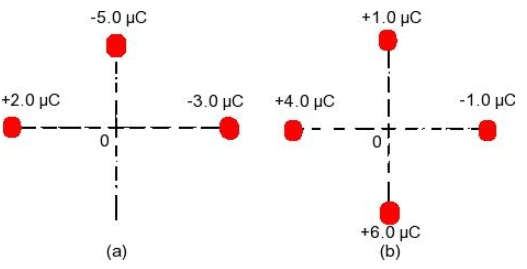# Problem: The drawing shows two situations in which charges are placed on the x and y axes. They are all located at the same distance of6.1 cm from the origin O. For each of the situations in the drawing, determine the magnitude of the net electric field at the origin.

###### FREE Expert Solution

Electric field:

$\overline{){\mathbf{E}}{\mathbf{=}}\frac{\mathbf{k}\mathbf{q}}{{\mathbf{r}}^{\mathbf{2}}}}$, where k is Coulomb's constant, q is the charge, and r is the distance from the point to the charge.

Vector magnitude:

$\overline{)\mathbf{|}\stackrel{\mathbf{⇀}}{\mathbit{A}}\mathbf{|}{\mathbf{=}}\sqrt{{{\mathbit{A}}_{\mathbit{x}}}^{\mathbf{2}}\mathbf{+}{{\mathbit{A}}_{\mathbit{y}}}^{\mathbf{2}}}}$

(a)

In this part, let's take:

q1 = 2.0μC = 2.0 × 10-6 C

q2 = -3.0μC = -3.0 × 10-6 C

q3 = - 5.0μC = -5.0 × 10-6 C

We calculate the x-component of the electric field as:

$\begin{array}{rcl}{\mathbf{E}}_{\mathbf{x}}& \mathbf{=}& \frac{\mathbf{k}{\mathbf{q}}_{\mathbf{1}}}{{{\mathbf{r}}_{\mathbf{1}}}^{\mathbf{2}}}\mathbf{+}\frac{\mathbf{k}{\mathbf{q}}_{\mathbf{2}}}{{{\mathbf{r}}_{\mathbf{2}}}^{\mathbf{2}}}\\ & \mathbf{=}& \mathbf{k}\mathbf{\left(}\frac{{\mathbf{q}}_{\mathbf{1}}}{{{\mathbf{r}}_{\mathbf{1}}}^{\mathbf{2}}}\mathbf{+}\frac{{\mathbf{q}}_{\mathbf{2}}}{{{\mathbf{r}}_{\mathbf{2}}}^{\mathbf{2}}}\mathbf{\right)}\\ & \mathbf{=}& \mathbf{\left(}\mathbf{9}\mathbf{.}\mathbf{0}\mathbf{×}{\mathbf{10}}^{\mathbf{9}}\mathbf{\right)}\mathbf{\left[}\frac{\mathbf{2}\mathbf{.}\mathbf{0}\mathbf{×}{\mathbf{10}}^{\mathbf{-}\mathbf{6}}}{{\mathbf{\left(}\mathbf{6}\mathbf{.}\mathbf{1}\mathbf{×}{\mathbf{10}}^{\mathbf{-}\mathbf{2}}\mathbf{\right)}}^{\mathbf{2}}}\mathbf{+}\frac{\mathbf{3}\mathbf{.}\mathbf{0}\mathbf{×}{\mathbf{10}}^{\mathbf{-}\mathbf{6}}}{{\mathbf{\left(}\mathbf{6}\mathbf{.}\mathbf{1}\mathbf{×}{\mathbf{10}}^{\mathbf{-}\mathbf{2}}\mathbf{\right)}}^{\mathbf{2}}}\mathbf{\right]}\end{array}$

84% (14 ratings)###### Problem Details

The drawing shows two situations in which charges are placed on the x and y axes. They are all located at the same distance of6.1 cm from the origin O. For each of the situations in the drawing, determine the magnitude of the net electric field at the origin.